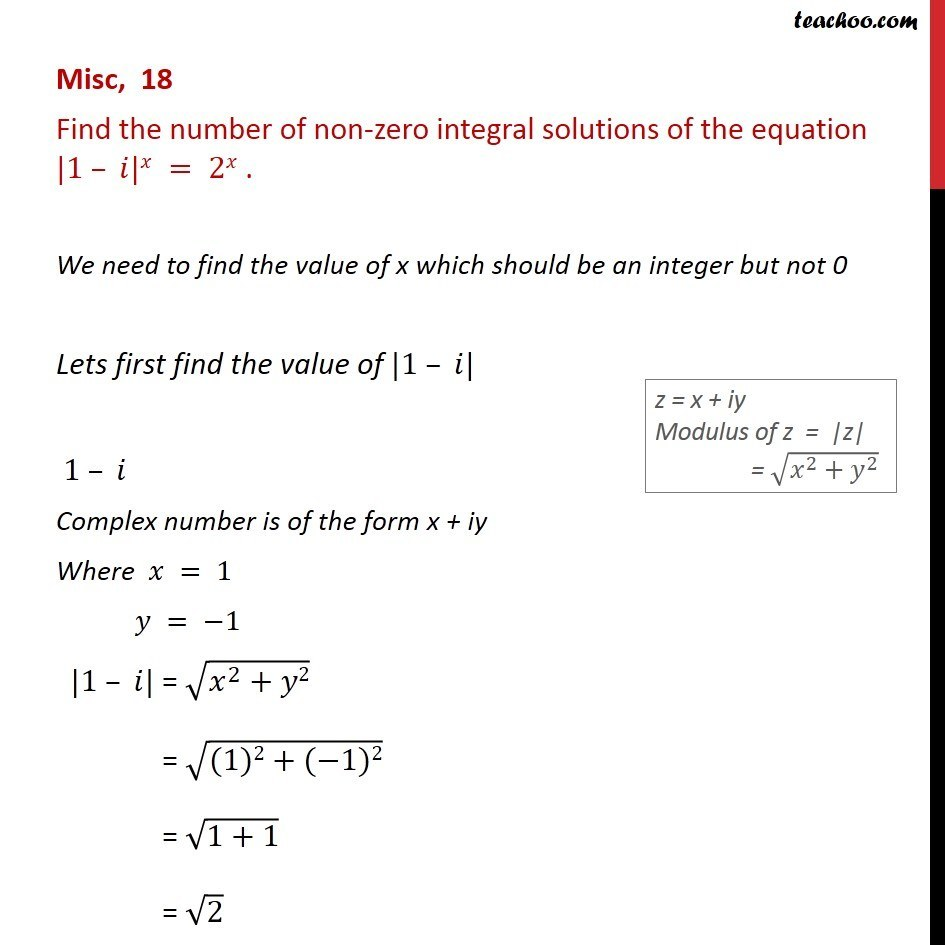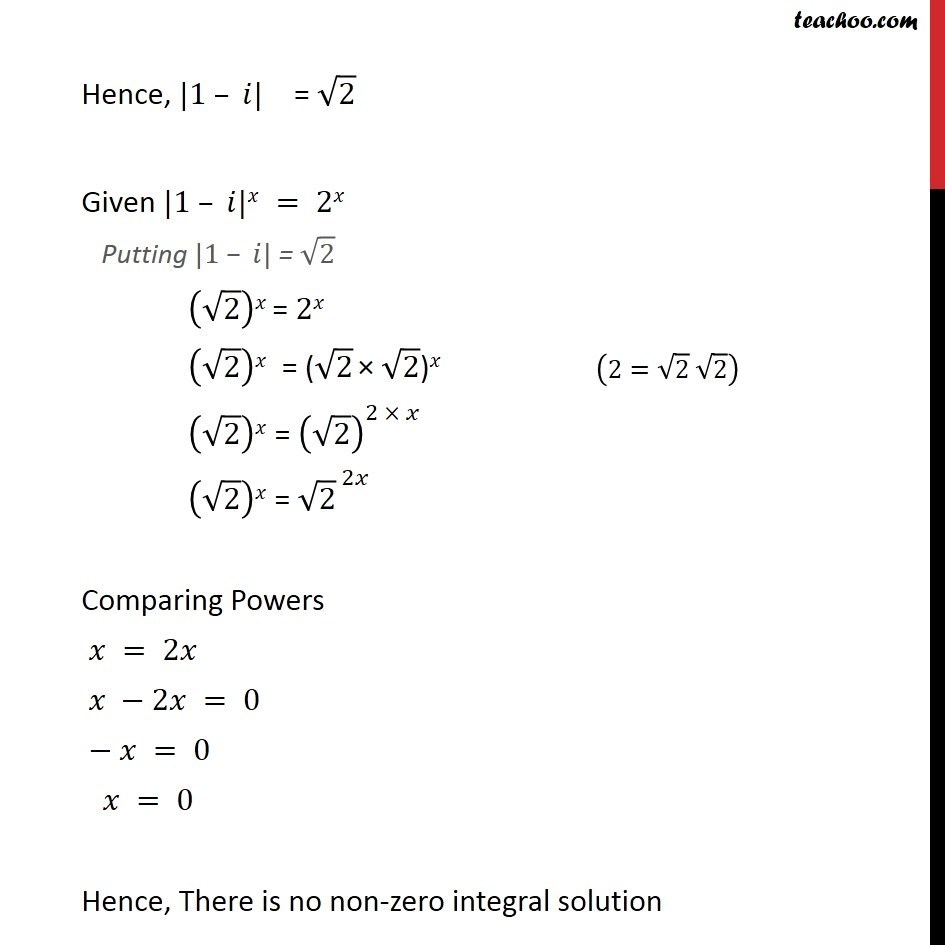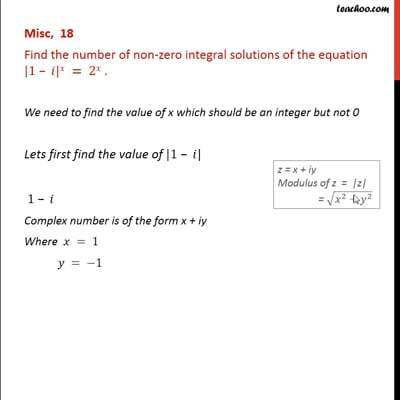Miscellaneous

Chapter 5 Class 11 Complex Numbers (Term 1)
Serial order wiseThis video is only available for Teachoo black users

### Transcript

Misc, 18 Find the number of non-zero integral solutions of the equation |1 – 𝑖|𝑥 = 2𝑥 . We need to find the value of x which should be an integer but not 0 Lets first find the value of |1 – 𝑖| 1 – 𝑖 Complex number is of the form x + iy Where 𝑥 = 1 𝑦 = −1 |1 – 𝑖| = √(𝑥^2+𝑦2) = √((1)2+(−1)2) = √(1+1) = √2 Hence, |1 – 𝑖| = √2 Given |1 – 𝑖|𝑥 = 2𝑥 Putting |1 – 𝑖| = √2 (√2)𝑥 = 2𝑥 (√2)𝑥 = (√2 × √2)𝑥 (√2)𝑥 = (√2)^(2 × 𝑥) (√2)𝑥 = 〖√2〗^( 2𝑥) Comparing Powers 𝑥 = 2𝑥 𝑥 −2𝑥 = 0 − 𝑥 = 0 𝑥 = 0 Hence, There is no non-zero integral solution